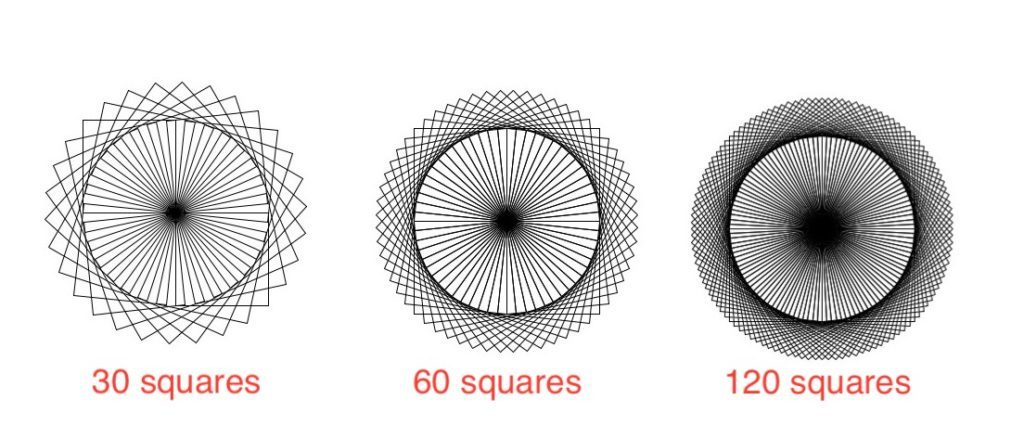# Circle of Squares using Python

Turtle library enables users to draw pictures or shapes using commands, providing them with a virtual canvas. turtle comes with Python’s Standard Library. It needs a version of Python with Tk support, as it uses tkinter for the graphics. In this article, we will generate a circular pattern out of squares using Python’s turtle module.

Examples:Pattern for different number of squares

Approach:

For drawing the circle, we need to draw n number of square, each time rotating the turtle cursor by d degrees. n and d are chosen such that `n*d=360`, so as to complete a full circle. In the implementation below we will draw a circle of 60 squares, rotating the cursor each time by 6 degrees.

Functions used:

• fd(x) : draw the cursor forward by x pixels.
• rt(x) : rotates the facing direction of the cursor by x degrees to the right.

Below is the implementation.

 `from` `turtle ``import` `*` ` `  ` `  `# loop for number of squares ` `for` `i ``in` `range``(``60``): ` `     `  `    ``# loop for drawing each square ` `    ``for` `j ``in` `range``(``4``): ` `         `  `        ``# drawing each side of ` `        ``# square of length 100  ` `        ``fd(``100``) ` `         `  `        ``# turning 90 degrees ` `        ``# to the right ` `        ``rt(``90``) ` `         `  `    ``# turning 6 degrees for ` `    ``# the next square ` `    ``rt(``6``) `

Output:

My Personal Notes arrow_drop_upCheck out this Author's contributed articles.

If you like GeeksforGeeks and would like to contribute, you can also write an article using contribute.geeksforgeeks.org or mail your article to contribute@geeksforgeeks.org. See your article appearing on the GeeksforGeeks main page and help other Geeks.

Please Improve this article if you find anything incorrect by clicking on the "Improve Article" button below.

Article Tags :

Be the First to upvote.

Please write to us at contribute@geeksforgeeks.org to report any issue with the above content.